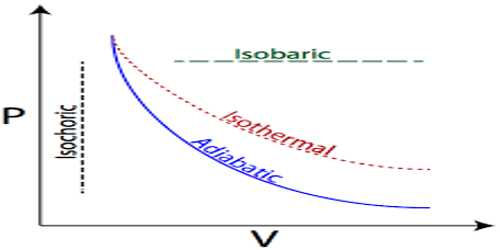Physics

# Use of First Law of Thermodynamics in Isothermal ProcessUse of the First Law of Thermodynamics in Isothermal process

The process in which temperature of a system remains constant but pressure and volume change it is called isothermal process. In this process, the internal energy of the system is not changed.

The first law of thermodynamics can be written as, dQ = du + dW …. ….. (1)

In isothermal process temperature remains constant; as a result, internal energy remains unchanged.

So, du = 0

Hence, equation (1) Can be written as, dQ = O + dW = dW … … … (2)

That means, in isothermal process work done by the system is equal to the heat supplied or absorbed by the system. Equation (2) is the mathematical form of the first law of thermodynamics in isothermal process.

Isothermal is the temperature is kept constant. A gas confined by a piston in a cylinder is again an example of this, only this time the gas is not heated or cooled, but the piston is slowly moved so that the gas expands or is compressed. The temperature is maintained at a constant value by putting the system in contact with a constant-temperature reservoir (the thermodynamic definition of a reservoir is something large enough that it can transfer heat into or out of a system without changing temperature).

If the volume increases while the temperature is constant, the pressure must decrease, and if the volume decreases the pressure must increase.

Example: A system rejects 500 J of heat at constant volume. Calculate the change in internal energy of the system. Explain the result.

dQ = du + dW = du + PdV

= – 500 J = du + 0; so, du = – 500 J

= – 500 J [Negative sign of internal energy means that the internal energy of the system decreases.)